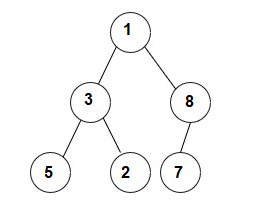New update is available. Click here to update.

# Postorder Successor

Posted: 2 Dec, 2020
Difficulty: Moderate

## PROBLEM STATEMENT

#### Note:

``````The postorder successor of ‘M’ is defined as the next element to ‘M’ in the sequence of postorder traversal.

If the postorder traversal of a tree is 3 5 4 7 then the postorder successor of 5 is the next element to 5 i.e 4.

Return ‘-1’ if there is no postorder successor of ‘M’.
``````
##### Input Format:
``````The first line contains an integer 'T' which denotes the number of test cases.

The first line of each test case contains elements of the tree in the level order form. The line consists of values of nodes separated by a single space. In case a node is NULL, we take -1 in its place.

The second line of each test case contains ‘M’, the node whose postorder successor is to be found out.

For example, the input for the tree depicted in the below image.
````````````1
3 8
5 2 7 -1
-1 -1 -1 -1 -1 -1
``````
##### Explanation :
``````Level 1 :
The root node of the tree is 1

Level 2 :
Left child of 1 = 3
Right child of 1 = 8

Level 3 :
Left child of 3 = 5
Right child of 3 = 2
Left child of 8 =7
Right child of 8 =  null (-1)

Level 4 :
Left child of 5 = null (-1)
Right child of 5 = null (-1)
Left child of 2 = null (-1)
Right child of 2 = null (-1)
Left child of 7 = null (-1)
Right child of 7 = null (-1)

The first not-null node(of the previous level) is treated as the parent of the first two nodes of the current level. The second not-null node (of the previous level) is treated as the parent node for the next two nodes of the current level and so on.
The input ends when all nodes at the last level are null(-1).
``````
##### Note :
``````The above format was just to provide clarity on how the input is formed for a given tree.
The sequence will be put together in a single line separated by a single space. Hence, for the above-depicted tree, the input will be given as:
1 3 8 5 2 7 -1 -1 -1 -1 -1 -1 -1
``````

#### Output Format:

``````Print an integer denoting the post-order successor of the node ‘M’ in the binary tree.

The output of each test case will be printed on a separate line.
``````

#### Note:

``````You do not need to print anything, it has already been taken care of. Just implement the given function.
``````

#### Constraints:

``````1<= ‘T’ <= 5
1 <= ‘N’ <= 3000
1 <= ‘M’ <= 3000
1 <= nodeVal <= 10^9

Time Limit: 1 sec
``````
SIMILAR PROBLEMS

Preorder Traversal

Posted: 18 Jan, 2022
Difficulty: Easy

Inorder Traversal

Posted: 19 Jan, 2022
Difficulty: Easy

Postorder Traversal

Posted: 20 Jan, 2022
Difficulty: Easy

Height of Binary Tree

Posted: 22 Apr, 2022
Difficulty: Easy

Locked Binary Tree

Posted: 12 May, 2022
Difficulty: Easy